• ## Python题目：学生信息管理系统

千次阅读 多人点赞 2019-11-18 20:17:14
Python题目：学生信息管理系统 选用list、tuple、dictionary或map等数据结构，存储X个学生的三门课的成绩（机器学习、Python程序设计、研究生英语），并实现以下功能： 1.添加学生信息 2.修改学生信息 3.删除...
Python题目：学生信息管理系统

选用list、tuple、dictionary或map等数据结构，存储X个学生的三门课的成绩（机器学习、Python程序设计、研究生英语），并实现以下功能：

1.添加学生信息
2.修改学生信息
3.删除学生
4.添加学生的成绩
5.修改学生成绩
6.按姓名或者学号查找学生，显示学生信息及三门课的成绩，以及排名
7.学生成绩统计（每门课的平均分、最高分、最低分）
现在把另一个高级版也放出来了，拥有图像界面显示，MySQL数据库操作：https://blog.csdn.net/kdongyi/article/details/103170876 -2019/11/20

stu_number = {
"182085211002":"张三",
"182085211003":"a",
"182085211004":"b",
"182085211005":"a",
"182085211006":"d",
"182085211007":"张三",
"182085211008":"f",
}

stu_info = [
["182085211002","张三","男","汉族","100","99","98"],
["182085211003","a","男","汉族","60","99","98"],
["182085211004","b","男","汉族","100","88","98"],
["182085211005","a","男","汉族","100","99","45"],
["182085211006","d","男","汉族","100","75","98"],
["182085211007","张三","男","汉族","100","75","98"],
["182085211008","f","男","汉族","50","60","60"],
]

rank = {}

# 添加学生信息
stu = []

if stu_id in stu_number.keys():  # 判断'stu_num'是否存在于stu_number的键中
print('该学生已存在，请勿重复添加！')
else:
stu_name = input("请输入学生姓名:")
stu_number[stu_id] = stu_name
stu.append(stu_id)
stu.append(stu_name)
stu.append(input("请输入学生性别:"))
stu.append(input("请输入学生民族:"))
stu.append("无")
stu.append("无")
stu.append("无")
stu_info.append(stu)

print_stu(stu_id)

# 修改学生信息
def modif_stu(stu):
if stu in stu_number.keys():  # 判断'stu_num'是否存在于stu_number的键中
print_stu(stu)
print("该学生信息如上，请输入要修改的项目:")
while True:
cmd = input("1、姓名  2、性别  3、民族 (输入q返回上级菜单):")
if cmd == 'q':
break
elif cmd == '1':
index = find_stu(stu)
for i in index:
stu_info[i] = input("请输入修改后的姓名:")
elif cmd == '2':
index = find_stu(stu)
for i in index:
stu_info[i] = input("请输入修改后的性别:")
elif cmd == '3':
index = find_stu(stu)
for i in index:
stu_info[i] = input("请输入修改后的民族:")
print("修改后的信息如下：")
print_stu(stu)

else:
print('该学生不存在，请添加或重新输入！')

# 删除学生
def delete_stu(stu):
if stu in stu_number.keys():  # 判断'stu_num'是否存在于stu_number的键中
print_stu(stu)
cmd = input("该学生信息如上，确定是否删除？(Y/N):")
if cmd == 'Y':
index = find_stu(stu)
for i in index:
del stu_info[i]
print("该学生已删除！")
else:
print('该学生不存在，请添加或重新输入！')

# 添加学生成绩
if stu_id in stu_number.keys():  # 判断'stu_num'是否存在于stu_number的键中
print_stu(stu_id)
print("该学生信息如上")

index = find_stu(stu_id)
for i in index:
stu_info[i] = input("请输入机器学习成绩:")
stu_info[i] = input("请输入Python程序设计成绩:")
stu_info[i] = input("请输入研究生英语:")
print("添加成绩结果如下：")
print_stu(stu)
else:
print('该学生不存在，请添加或重新输入！')

# 修改学生成绩
def modif_stu_val(stu):
if stu in stu_number.keys():  # 判断'stu_num'是否存在于stu_number的键中
print_stu(stu)
print("该学生信息如上，请输入要修改的课程:")
while True:
cmd = input("1、机器学习  2、Python程序设计  3、研究生英语 (输入q返回上级菜单):")
if cmd == 'q':
break
elif cmd == '1':
index = find_stu(stu)
for i in index:
stu_info[i] = input("请输入修改后的成绩:")
elif cmd == '2':
index = find_stu(stu)
for i in index:
stu_info[i] = input("请输入修改后的成绩:")
elif cmd == '3':
index = find_stu(stu)
for i in index:
stu_info[i] = input("请输入修改后的成绩:")
print("修改后的信息如下：")
print_stu(stu)

else:
print('该学生不存在，请添加或重新输入！')

# 排名
def ranking():
to_rank = {}
for stu in stu_info:
if stu=='无' or stu=='无' or stu=='无':
to_rank[stu] = 0
rank[stu] = '无'
else:
to_rank[stu] = (int(stu)+int(stu)+int(stu))/3
ranked = sorted(to_rank.items(),key = lambda x:x,reverse = True)
num = 1
re_num = 0 #分一样
for i in range(len(ranked)):
if i!=0:
if ranked[i]==ranked[i-1]: #考虑分数一样的情况 排名相同
re_num+=1
rank[ranked[i]] = num
else:
num = num + 1 + re_num #分数不一样 排名加一 如果有重复排名则加上
rank[ranked[i]] = (num + re_num)
re_num = 0
else: #存第一名
rank[ranked[i]] = num

# 按姓名或者学号查找学生，返回值为列表 列表里的值为该学生数据位置
# 如果有重名学生，则返回在列表返回多个学生
def find_stu(stu):
stu_index = []
for i in stu_info:
try:
if i.index(stu) != None: #如果能查找到
stu_index.append(stu_info.index(i))
except:pass
return stu_index

# 根据姓名或者学号显示学生信息及三门课的成绩、排名
def print_stu(stu):
ranking()  # 排名
stu_index = find_stu(stu)
if len(stu_index) == 0:
print("无此学生，请核对后重新输入！")
for i in stu_index:
the_stu = stu_info[i]
print("\t姓名:",the_stu)
print("\t学号:", the_stu)
print("\t性别:", the_stu)
print("\t民族:", the_stu)
print("\t机器学习成绩:", the_stu)
print("\tPython程序设计成绩:", the_stu)
print("\t研究生英语成绩：:", the_stu)
print("\t排名：:", rank[the_stu])
print("--------------------------")

def statistics():
machine_learning_max = 0
python_max = 0
english_max = 0
machine_learning_min = 100
python_min = 100
english_min = 100
machine_learning = 0
python = 0
english = 0
num = 0

for stu in stu_info:
if stu!='无' and stu!='无' and stu!='无':
num += 1
machine_learning += float(stu)
python += float(stu)
english += float(stu)

if float(stu) > machine_learning_max: machine_learning_max = float(stu)
if float(stu) < machine_learning_min: machine_learning_min = float(stu)
if float(stu) > python_max: python_max = float(stu)
if float(stu) < python_min: python_min = float(stu)
if float(stu) > english_max: english_max = float(stu)
if float(stu) < english_min: english_min = float(stu)

machine_learning = machine_learning/num
python = python/num
english = english/num

print("学生成绩统计如下：")
print("机器学习：")
print("\t平均分：%.2f,最高分：%.2f,最低分：%.2f" % (machine_learning,machine_learning_max,machine_learning_min))
print("Python程序设计：")
print("\t平均分：%.2f,最高分：%.2f,最低分：%.2f" % (python, python_max, python_min))
print("研究生英语：")
print("\t平均分：%.2f,最高分：%.2f,最低分：%.2f" % (english, english_max, english_min))

while True:
print("--------------------------")
print("请输入以下命令进行操作：")
print("\t1 添加学生信息")
print("\t2 修改学生信息")
print("\t3 删除学生")
print("\t4 添加学生的成绩")
print("\t5 修改学生成绩")
print("\t6 按姓名或者学号查找学生，显示学生信息及三门课的成绩，以及排名")
print("\t7 学生成绩统计")
print("\texit 退出系统！")
cmd = input("请输入命令:")

if cmd == 'exit': # 退出系统！
break
elif cmd == '1': # 添加学生信息
print("--------------------------")
while True:
stu_id = input("请输入要添加学号(输入q返回上级菜单):")
if stu_id == 'q':
break

elif cmd == '2': # 修改学生信息
print("--------------------------")
while True:
stu = input("请输入要修改的学生学号(输入q返回上级菜单):")
if stu == 'q':
break
modif_stu(stu)

elif cmd == '3': # 删除学生
print("--------------------------")
while True:
stu = input("请输入要删除的学生学号(输入q返回上级菜单):")
if stu == 'q':
break
delete_stu(stu)

elif cmd == '4': # 添加学生的成绩
print("--------------------------")
while True:
stu = input("请输入要添加成绩的学号(输入q返回上级菜单):")
if stu == 'q':
break

elif cmd == '5': # 修改学生成绩
print("--------------------------")
while True:
stu = input("请输入要修改成绩的学号(输入q返回上级菜单):")
if stu == 'q':
break
modif_stu_val(stu)

elif cmd == '6': # 按姓名或者学号查找学生，显示学生信息及三门课的成绩，以及排名
ranking() # 排名
print("--------------------------")
while True:
stu = input("请输入要查询的学生姓名或学号(输入q返回上级菜单):")
if stu == 'q':
break
print_stu(stu)

elif cmd == '7': # 学生成绩统计
print("--------------------------")
statistics()




展开全文学生信息管理系统
• Python题目：个人所得税计算 rate = [0.03, 0.1, 0.2, 0.25, 0.3, 0.35, 0.45] quict_cal = [0, 210, 1410, 2660, 4410, 7160, 15160] salary = float(input("请输入您的月工资：")) if salary <= 5000: ...
Python题目：个人所得税计算

rate = [0.03, 0.1, 0.2, 0.25, 0.3, 0.35, 0.45]
quict_cal = [0, 210, 1410, 2660, 4410, 7160, 15160]
salary = float(input("请输入您的月工资："))

if salary <= 5000:
print("恭喜您，您的工资在免征税范围内！")
elif salary > 5000 and salary <= 8000:
print("使用税率：",rate)
print("您应该缴纳的个人所得税为：%.3f元" % (salary * rate - quict_cal))
elif salary > 8000 and salary <= 17000:
print("使用税率：", rate)
print("您应该缴纳的个人所得税为：%.3f元" % (salary * rate - quict_cal))
elif salary > 17000 and salary <= 30000:
print("使用税率：", rate)
print("您应该缴纳的个人所得税为：%.3f元" % (salary * rate - quict_cal))
elif salary > 30000 and salary <= 40000:
print("使用税率：", rate)
print("您应该缴纳的个人所得税为：%.3f元" % (salary * rate - quict_cal))
elif salary > 40000 and salary <= 60000:
print("使用税率：", rate)
print("您应该缴纳的个人所得税为：%.3f元" % (salary * rate - quict_cal))
elif salary > 60000 and salary <= 85000:
print("使用税率：", rate)
print("您应该缴纳的个人所得税为：%.3f元" % (salary * rate - quict_cal))
elif salary > 85000:
print("使用税率：", rate)
print("您应该缴纳的个人所得税为：%.3f元" % (salary * rate - quict_cal))



展开全文个人所得税计算
• ## 经典python题目练习

万次阅读 多人点赞 2017-09-12 11:49:41
1、给定一个非空正整数的.../usr/bin/python list = [1,1,1,6,6,7,3,9] a = {} for i in list: if list.count(i)>0: a[i] = list.count(i) #a[i]是字典value的值 print a # items()方法将字典的元素转化为了元...
1、给定一个非空正整数的数组，按照数组内数字重复出现次数，从高到低排序

#!/usr/bin/python
list = [1,1,1,6,6,7,3,9]
a = {}
for i in list:
if list.count(i)>0:
a[i] = list.count(i)  #a[i]是字典value的值

print a
# items()方法将字典的元素转化为了元组
# key参数对应的lambda表达式的意思则是选取元组中的第二个元素作为比较参数
# lambda x:y中x表示输出参数，y表示lambda函数的返回值
b = sorted(a.items(),key=lambda item:item)
#逆转次数从大到小排序
b.reverse()
print b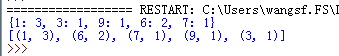2、输入输出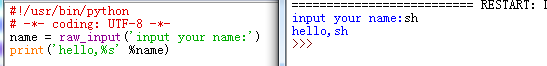3、输出格式多样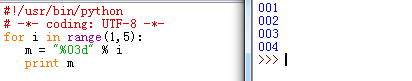4、list.append(i);list.sort()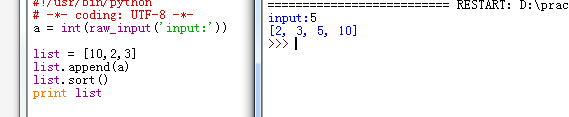5、n = m[:]   列表n复制列表m全部数据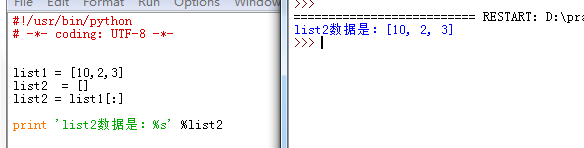6、time.sleep(1)# 暂停 1 秒

7、a%b==0 表示能被整除；a/b 获得相除后的值（非余数）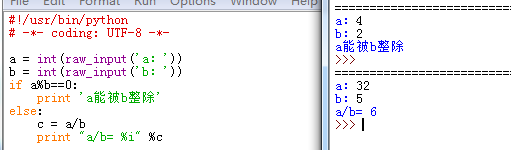8、列表组合后抛开重复值，排序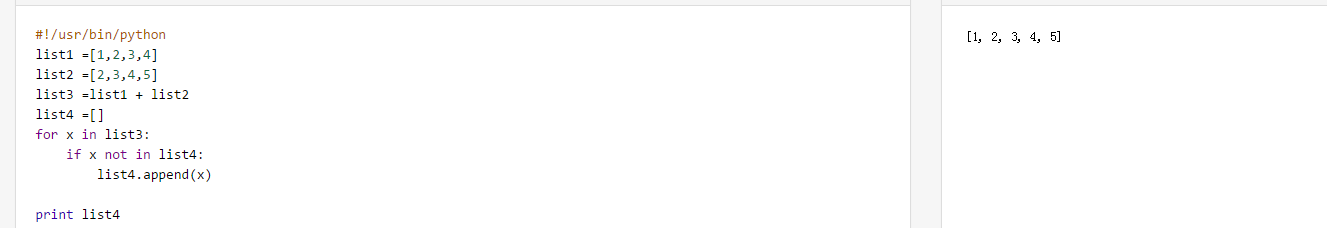9、判断是否字符、数字、空格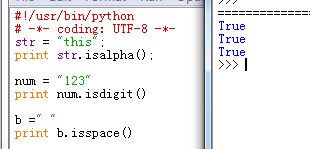10、求列表组合值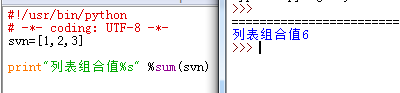11、1!+2!+3!的和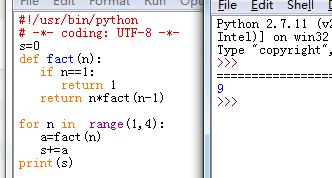12、递归调用，自定义方法，实现倒序字符串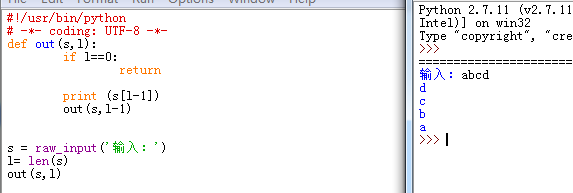13、python切片

a = ['one', 'two', 'three']print a

结果：one

************************

a = ['one', 'two', 'three']print a[0:2]

结果：one,two(显示序号2 之前的数据)

*************************

a = ['one', 'two', 'three']
print a[::-1]

结果：three,two,one(数据倒序)

**************************

a = ['one', 'two', 'three']
print a[::2]

结果：one，three(间隔显示数据)

14、函数调用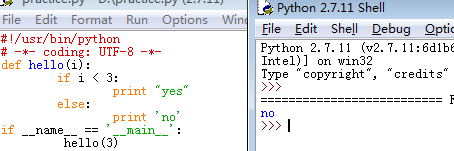15、排序操作 sort（），reverse()

b = sorted(a)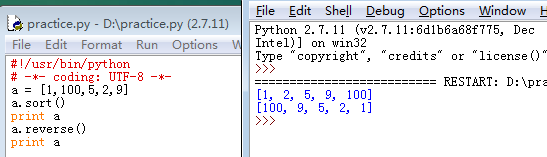16、插入数值排序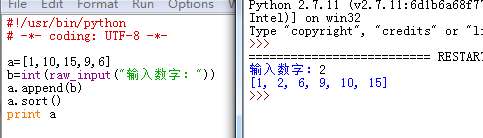17、变量作用域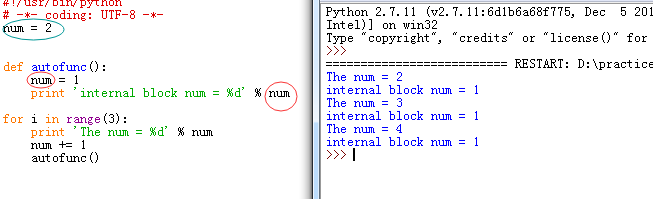18、嵌套列表相加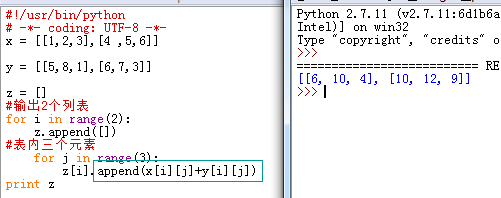19、变量值互换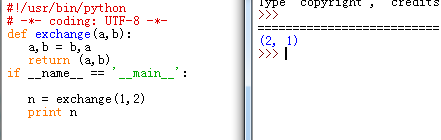20、计算字符串长度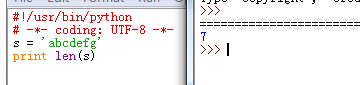21、列表中最小和最大值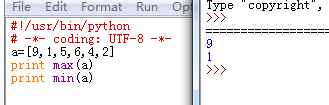22、连接两个表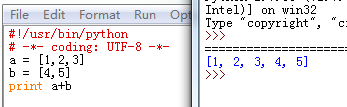23、 字典最大值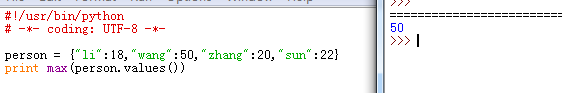24、列表生成式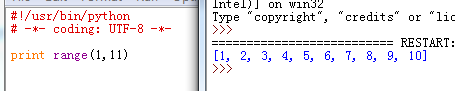25、生成器

在Python中，这种一边循环一边计算的机制，称为生成器（Generator）

方法一：把一个列表生成式的[]改成()，就创建了一个generator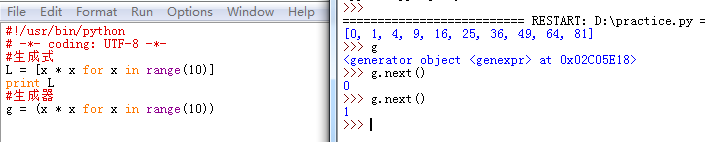方法二：通过特殊函数创建：如果一个函数定义中包含yield关键字，那么这个函数就不再是一个普通函数，而是一个generator

例如：递归斐波那契数列

1、普通函数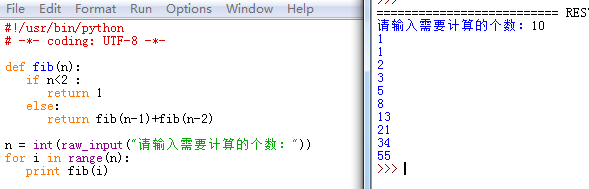2、generator方式：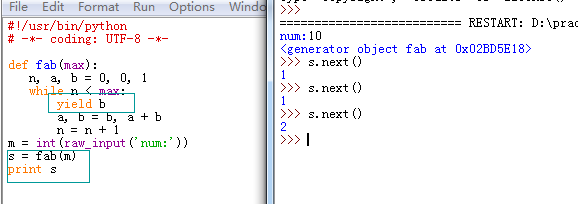ps:函数是顺序执行，遇到return语句或者最后一行函数语句就返回。而变成generator的函数，在每次调用next()的时候执行，遇到yield语句返回，再次执行时从上次返回的yield语句处继续执行。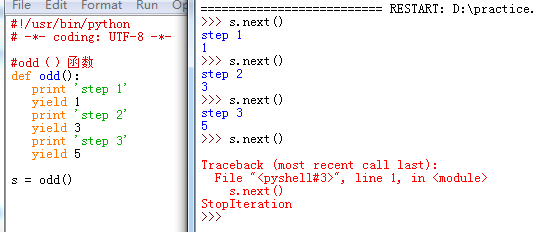26、迭代器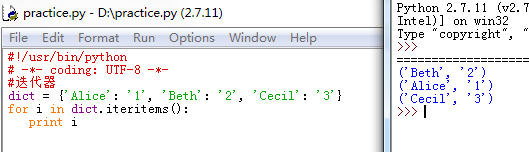展开全文• ## python题目(1)

千次阅读 2020-05-04 14:47:07
1．Python语言属于（ ）。C A． 机器语言 B． 汇编语言 C．高级语言 D．科学计算语言 2．下列选项中，不属于Python特点的是（ ）。B A．面向对象 B．运行效率高 C．可读性好 D．开源 3．Python程序文件的扩展名是...
一、选择题
1．Python语言属于（      ）。C
A． 机器语言      B． 汇编语言      C．高级语言       D．科学计算语言
2．下列选项中，不属于Python特点的是（      ）。B
A．面向对象       B．运行效率高     C．可读性好       D．开源
3．Python程序文件的扩展名是（      ）。D
A．.python        B．.pyt           C．.pt            D．.py
4．以下叙述中正确的是（     ）。C
A．Python 3.x与Python 2.x兼容
B．Python语句只能以程序方式执行
C．Python是解释型语言
D．Python语言出现得晚，具有其他高级语言的一切优点
5．下列选项中合法的标识符是（      ）。A
A．_7a_b             B．break          C．_a\$b             D．7ab
6．下列标识符中合法的是（      ）。B
A．i'm              B．_              C．3Q            D．for
7．Python不支持的数据类型有（      ）。A
A．char	     	B．int  	    	C．float 	    	D．list
8．关于Python中的复数，下列说法错误的是（      ）。B
A．表示复数的语法形式是a+bj           B．实部和虚部都必须是浮点数
C．虚部必须加后缀j，且必须是小写      D．函数abs()可以求复数的模
9．函数type(1+0xf*3.14)的返回结果是（      ）。D
A．<class 'int'>             B． <class 'long'>
C．<class 'str'>             D． <class 'float'>
10．字符串s='a\nb\tc'，则len(s)的值是（      ）。C
A．7            B．6       C．5                D．4
11．Python语句print(0xA+0xB)的输出结果是（      ）。D
A．0xA+0xB                         B．A+B
C．0xA0xB                          D．21
12．下列属于math库中的数学函数的是（      ）。C
A．time()	     B．round()        C．sqrt()      D．random()
13．Python表达式中，可以使用（      ）控制运算的优先顺序。A
A．圆括号()       B．方括号[]        C．大括号{}     D．尖括号<>
14．下列表达式中，值不是1的是（      ）。D
A．4//3           B．15 % 2        C．1^0          D．~1
15．Python语句print(r"\nGood")的运行结果是（      ）。C
A．新行和字符串Good             B．r"\nGood"
C．\nGood                        D．字符r、新行和字符串Good
16．语句eval('2+4/5')执行后的输出结果是（      ）。A
A．2.8	           B．2          C．2+4/5	    	D．'2+4/5'
17．整型变量x中存放了一个两位数，要将这个两位数的个位数字和十位数字交换位置，例如，13变成31，正确的Python表达式是（      ）。A
A．（x%10）*10+x//10            B．(x%10)//10+x//10
C．(x/10)%10+x//10              D．(x%10)*10+x%10
18．与数学表达式 对应的Python表达式中，不正确的是（      ）。C
A．c*d/(2*a*b)            B．c/2*d/a/b
C．c*d/2*a*b              D．c*d/2/a/b

二、填空题
1．Python语句既可以采用交互式的____执行方式，又可以采用 ___执行方式。


命令、程序

2．在Python集成开发环境中，可使用快捷键__运行程序。


F5

3.使用math模块库中的函数时，必须要使用        语句导入该模块。


import math

4．Python表达式1/2的值为        ，1//3+1//3+1//3的值为        ，5%3的值为        。


0.5，0，2

5．Python表达式0x66 & 0o66的值为        。


38

6．设m，n为整型，则与m%n等价的表达式为        。


m-m//n*n

7．计算231-1的Python表达式是        。


2**31-1 或 (1<<31)-1

8．数学表达式 的Python表达式为       。


math.exp(abs(x-y))/(pow(3,x)+math.sqrt(6)*math.sin(y))

三、问答题
1．Python语言有何特点？


2．简述Python的主要应用领域及常用的函数模块。


3．Python语言有哪些数据类型？


4．写出下列数学式对应的Python表达式。
（1）        		（2）


5．按要求写出Python表达式。
（1）将整数k转换成实数。
（2）求实数x的小数部分。
（3）求正整数m的百位数字。
（4）随机产生一个8位数，每位数字可以是1到6中的任意一个整数。


6．下列语句的执行结果是False，分析为什么?

from math import sqrt
print(sqrt(3)*sqrt(3)==3)
False

具体word文件可以去我的资源中下载


展开全文• ## python题目-完数

千次阅读 2019-02-26 14:05:32
题目: 一个数如果恰哈等于它的因子之和，这个数被称为'完数' 如6=1+2+3.找出1000以内的所有完数       from functools import reduce d = [] for a in range(2,1001): c = [] for b in range(1,a): ...python
• ## python题目-判断素数

千次阅读 2019-02-25 10:22:50
题目: #判断101-200之间有多少个素数,并输出所有素数 #一个大于1的正整数，如果除了1和它本身以外，不能被其他正整数整除，就叫素数。如2，3，5，7，11，13，17. b = [] for i in range(101, 201): for a in ...python
• ## python题目-成绩分类

千次阅读 2019-02-25 16:30:18
题目: 利用条件运算符的嵌套来完成此题 学习成绩&gt;=90分的同学用A表示，60-89分之间的用B表示，60分下的用C表示     score = int(input('此次的学习成绩为')) if score &gt;= 90: print('此同学...python
• 题目; 输入任意一个数字计算阶乘 a = int(input('请任意输入一个数字的阶乘')) b = 1 if a &lt; 0: print('负数没有阶乘!') elif a == 0: print('0的阶乘固定为1!') else: for i in range(1,a+1): b =...python
• 题目: 一个5位数，判断它是不是回文数. 即12321是回文数，个位与万位相同，十位与千位相同 try: str = int(input('输入任意5位数:')) if len(str) &gt; 5: print('输入数字长度错误！') else: if ...python
• 题目: 一只青蛙从井底往井口跳，每天跳一次，每次跳3米，下滑1米，井口99米 第几次可以跳出井口(边缘也算) 任意高度井口   a = input('输入井口高度:') b = int(a) / 2 if int(list(str(b))[-1]) &gt; 1: ...python
• 题目: 有一对兔子，从出生后第3个月起每个月都生一对兔子 小兔子长到第三个月后每个月又生一对兔子 假如兔子都不死，问每个月的兔子总数为多少   代码: import math for a in range(1,51): b = math.pow(2...python
• 题目 分别构造22-25，32~35，42-45，52-55共16个矩阵 在屏幕端可以选择打印相关矩阵 程序运行后，只需提示有哪些类型的矩阵即可 实现矩阵运算，包括矩阵的乘法和矩阵的转置，在屏幕端选择运算类型以及需要进行运算...
• 某个公司采用公用电话传递数据，数据是四位的整数，在传递过程中是加密的 加密规则如下:每位数字都加上5，然后用和除以10的余数代替该数字，再将第一位和第四位交换，第二位和第三位交换 ...python
• 打印出100-999所有的水仙花数，所谓水仙花数是指一个三位数，其各位数字立方和等于该数本身 如:153是一个'水仙花数'，因为153=1的三次方+5的三次方+3的三次方 n = [] for i in range(100,1000): ...python
• 看到这个题目时，可以分析每一行字符的组成规律，就可以分成上下两部来实现。实现的代码如下：#python 3.6 import sys print('请输入要打印的字符: ') printChar = input() print('请输入层数: ') count = input()...tensorflow
• 本人用的是python3.7 ，有道接口帮助文档用有 python2.0获取坐标的demo 案例完整代码： #!/usr/bin/env python # -*- coding: utf-8 -*- # @version : Python 3.7.3 # @Time : 2019/8/15 17:20 import uuid ...
• 题目: 有一分数序列:2/1,3/2,5/3,8/5,13/8,21/13 求出这个数列的前20项之和     from functools import reduce a = 2 b = 1 i = [] for n in range(1,21): c = a / b a,b = a + b,a i.append(c) m = ...python
• 题目: 请输入星期几的第一个字母来判断一下是星期几 如果第一个字母一样，则继续判断第二个字母 x = input('请输入第一位小写字母:') if x == 'm': print('星期一') elif x == 't': b = input('请...python
• 编写一个函数，输入n为偶数时，调用函数求1/2+1/4+...+1/n 当输入n为奇数时，调用函数1/1+1/3+...+1/n from functools import reduce a = [] c = [] ... for i in range(1,n+1): ... a.append(...python
• Python中,无论是对象赋值，作为为参数传递,作为函数返回值,都是引用传递的.如果需要拷贝对象，需要使用标准库中的copy模块（import copy）。 1.浅拷贝 ：  使用copy.copy,它可以进行对象的浅拷贝(shallow ...
• 题目：输入一个整数N，输出N的所有最小因子，也称素因子。其中，任何一个大于1的数,都可以写成多个素数的乘积,我们把这些素数叫做这个数素因子。例如：输入：120输出：2 2 2 3 5输入：27输出：3 3 3python求解素因子...
• 1、 2、 3、 4、 5、 6、 7、 8、 9、 10、 11、 12、 13、 14、 15、 16、 17、 18、计算机二级
• 求出满足下列条件的4位数： 1、该数是完全平方数 2、且第一位和第三位数字之和为10 3、第二位与第四位数字之积为12 import math for num in range(1000,10000): #生成4位数 if len(str(math.sqrt(num))) == 4...
• A+B Problem Problem Description Calculate a+b. Input Two integer a,b (0&lt;=a,b&lt;=10). Output Output a+b. Sample Input 1 2 Sample ......

# python题目python 订阅